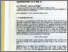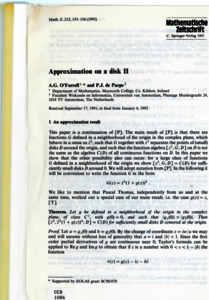# Approximation on a disk II

O'Farrell, A.G. and de Paepe, P.J. (1993) Approximation on a disk II. Mathematische Zeitschrift (212). pp. 153-156. ISSN 1432-1823Previewmore...Add this article to your Mendeley library

## Abstract

This paper is a continuation of [P]. The main result of [P] is that there are functions G defined in a neighborhood of the origin in the complex plane, which behave in a sense as z2, such that G together with z 2 separates the points of (small) disks D around the origin, and such that the function algebra [z2, G; D] on Dis not the same as the algebra C(D) of all continuous functions on D. In this paper we show that the other possibility also can occur: for a large class of functions G defined in a neighborhood of the origin we show [z2 , G; D] = C(D) for sufficiently small disks D around 0. We will adopt notation from [P]. In the following it will be convenient to write the function G in the form G(z) = z2 (1 + g(z))2 We like to mention that Pascal Thomas, independently from us and at the same time, worked out a special case of our main result, i.e. the case g(z) = z, [T].

Item Type: Article Approximation; Disk; Faculty of Science and Engineering > Mathematics and Statistics 14688 https://doi.org/10.1007/BF02571648 Prof. Anthony O'Farrell 11 Aug 2021 13:33 Mathematische Zeitschrift Springer Berlin / Heidelberg YesItem control page